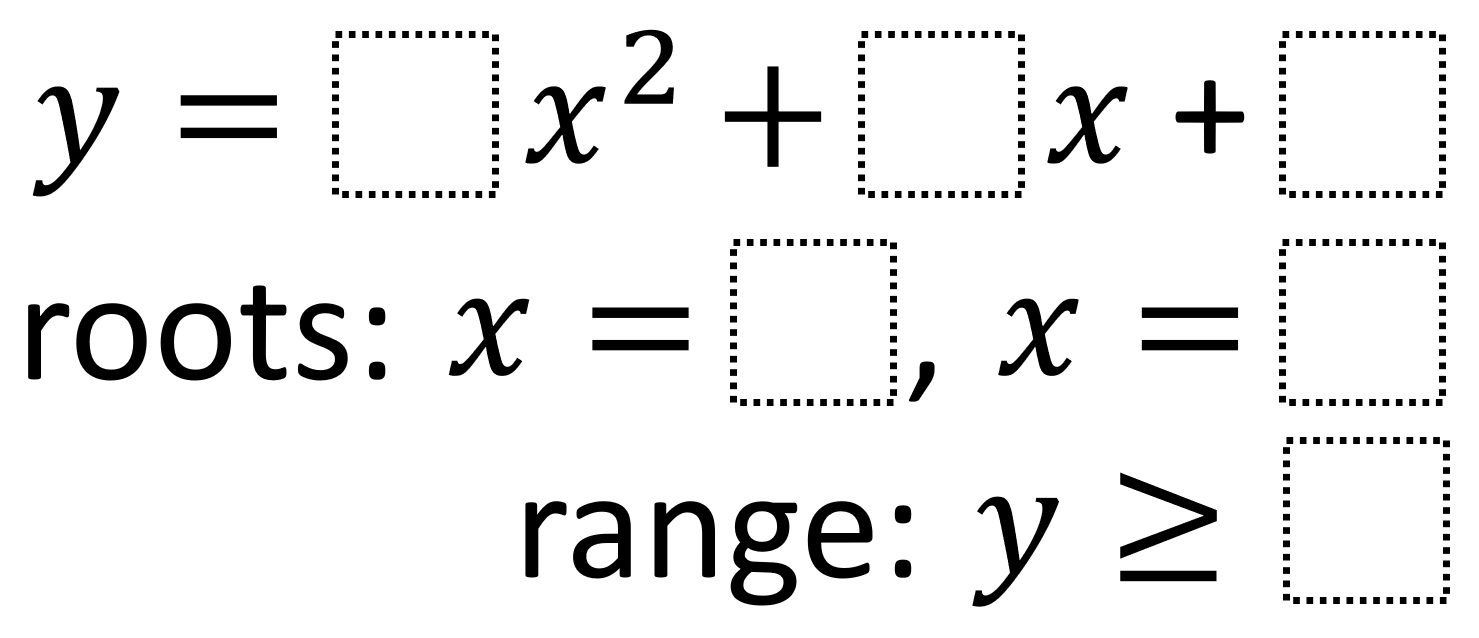# Trinomial Function Features 1

Directions: Using the integers -9 to 9, at most one time each, place an integer in each box to create a function with the corresponding range and roots.### Hint

How do each of the values you can choose affect the values of the roots and range?

There are many possible answers but all will have a minimum and point upwards including:

y = 1x^2 + 6x + 8
roots: x = -4 and x = -2
range: y ≥ -1

y = 1x^2 + 6x + 5
roots: x = -5 and x = -1
range: y ≥ -4

Source: Robert Kaplinsky

## Period of Trig Function 3

Directions: Using the digits 0 to 9, at most one time, fill in the blanks …